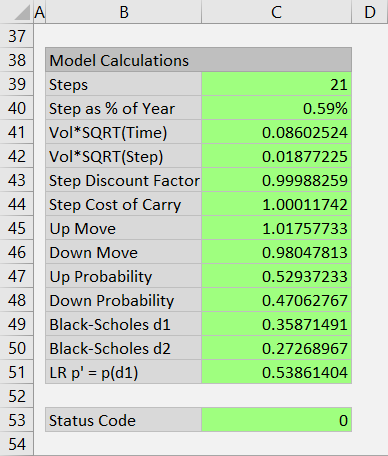# Leisen-Reimer Model

This page explains the implementation of Leisen-Reimer model in the Binomial Option Pricing Calculator.

All three models supported by the calculator – this one, Cox-Ross-Rubinstein and Jarrow-Rudd – follow the same logic for constructing binomial trees (that part is explained in underlying price tree and option price tree).

The models only differ in sizes and probabilities of underlying price up and down moves in the underlying price tree. Leisen-Reimer calculations of these are explained below.

## Main Idea of Leisen-Reimer Model

Each model has some basic idea – a special characteristic of its underlying price tree.

In Jarrow-Rudd model, probabilities of up and down moves are always the same (50%). The Jarrow-Rudd tree is therefore symmetrical in terms of probabilities.

In Cox-Ross-Rubinstein model, one up move and one down move always result in the same price (UpMove * DownMove = 1), which makes the CRR tree symmetrical in terms of prices and centered around the initial underlying price.

Leisen-Reimer trees are not symmetrical in terms of either prices or probabilities, but they have another very useful feature: At expiration (the final step), prices are centered around the option's strike price.

This makes the Leisen-Reimer model typically more accurate with fewer number of steps compared to the other two models – with one limitation: number of steps must be odd. Another downside is that Leisen-Reimer calculations of move sizes and probabilities are a bit more complicated – but not too much, as we will show below.

## Calculations in the Main Sheet

In the calculator, you can find these calculations in the Model Calculations section at the bottom of the Main sheet, in cells B38-C51.Some formulas are the same for all models, but most are different. Those are implemented with Excel IF functions. The id of Leisen-Reimer model in the calculator is 3, so the relevant part of the IF formulas is always the one where the condition Model=3 is true.

As mentioned above, Leisen-Reimer model requires an odd number of steps. Even steps don't produce errors – the calculations can still be made, but the resulting option price is much less precise.

Therefore, by default, when even number of steps in entered (in cell C4) and Leisen-Reimer model is selected (dropdown box in cell C3), the calculator automatically adjusts the number of steps to the next higher odd number (e.g. 20 steps becomes 21, while 21 stays 21). This is done in cell C39.

If you, for some reason, want to use Leisen-Reimer model with even number of steps, you can switch off this automatic adjustment by changing the LRAdjustSteps setting to FALSE in the Preferences sheet.

## Up and Down Move Probabilities

Leisen-Reimer calculations start from up move probability, which is in cell C47, named UpProb. The Leisen-Reimer part of this formula is at the end – it is the third argument of the last IF function.

UpProb = 0.5+SIGN(BS_d2)/2*SQRT(1-EXP(-((BS_d2/(Steps+1/3+0.1/(Steps+1)))^2*(Steps+1/6))))

It is long and looks complicated, but it is composed of basic Excel functions only: SIGN, SQRT and EXP. The entire expression is the Peizer-Pratt binomial distribution inversion formula, which takes only two arguments:

Steps = (adjusted) number of steps in cell C39

BS_d2 = the d2 which you may know from the Black-Scholes model, here calculated in cell C50

Note: Out of the two alternative Peizer-Pratt inversion methods, this calculator uses the longer and more precise one, sometimes denoted PP2. The difference is only the 0.1/(Steps+1) part, which the other method (PP1) does not include.

Probability of down move (cell C48) is of course:

DownProb = 1 – UpProb

... because in binomial trees, the two probabilities must add up to 1.

## Up and Down Move Sizes

Once we have the probabilities, we can calculate the sizes of up and down moves, which are in cells C45 (UpMove) and C46 (DownMove). The Leisen-Reimer version is again the last part of these formulas where Model=3 condition is true.

UpMove = StepCost * LR_p_d1 / UpProb

DownMove = StepCost * (1-LR_p_d1) / (1-UpProb)

StepCost is calculated in cell C44:

StepCost = EXP((IntRate-Yield)*StepPct)

IntRate = interest rate input in cell C26

Yield = dividend yield (for stock or index options) or second interest rate (for currency options) in cell C29

StepPct = duration of one step as % of year = time to expiration / number of steps

LR_p_d1 is the already familiar Peizer-Pratt inversion, this time for Black-Scholes d1, calculated in cell C51:

LR_p_d1 = 0.5+SIGN(BS_d1)/2*SQRT(1-EXP(-((BS_d1/(Steps+1/3+0.1/(Steps+1)))^2*(Steps+1/6))))

Now we have calculated the sizes and probabilities of up and down moves, which is all we need for building the underlying price tree and option price tree.

By remaining on this website or using its content, you confirm that you have read and agree with the Terms of Use Agreement.

We are not liable for any damages resulting from using this website. Any information may be inaccurate or incomplete. See full Limitation of Liability.

Content may include affiliate links, which means we may earn commission if you buy on the linked website. See full Affiliate and Referral Disclosure.

We use cookies and similar technology to improve user experience and analyze traffic. See full Cookie Policy.